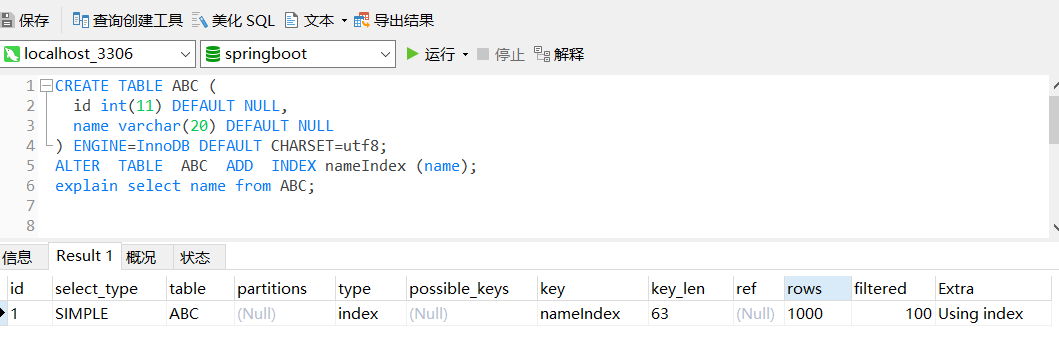# varchar(20)和varchar(255)的区别，数据库表字段长度设计学习# 验证varchar(20)和varchar(255)的区别

``````CREATE TABLE ABC (
id int(11) DEFAULT NULL,
name varchar(20) DEFAULT NULL
) ENGINE=InnoDB DEFAULT CHARSET=utf8; -- 创建表
ALTER  TABLE  ABC  ADD  INDEX nameIndex (name);  -- 给name 字段添加索引
explain select name from ABC;    --  分析这个查询sql语句
````````````SELECT CONCAT(TRUNCATE(SUM(data_length),2),'B') AS data_size,
CONCAT(TRUNCATE(SUM(max_data_length),2),'B') AS max_data_size,
CONCAT(TRUNCATE(SUM(data_free),2),'B') AS data_free,
CONCAT(TRUNCATE(SUM(index_length),2),'B') AS index_size
FROM information_schema.tables WHERE TABLE_NAME = 'ABC';
````````````alter table ABC  modify name varchar(255);
explain select name from ABC;
SELECT CONCAT(TRUNCATE(SUM(data_length),2),'B') AS data_size,
CONCAT(TRUNCATE(SUM(max_data_length),2),'B') AS max_data_size,
CONCAT(TRUNCATE(SUM(data_free),2),'B') AS data_free,
CONCAT(TRUNCATE(SUM(index_length),2),'B') AS index_size
FROM information_schema.tables WHERE TABLE_NAME = 'ABC';
``````

KEY_LEN变成了768 但是data_size没有变化。

# 额外的发现

### 评论